StatLect

# Multicollinearity

Multicollinearity is a problem that affects linear regression models in which one or more of the regressors are highly correlated with linear combinations of other regressors. When this happens, the OLS estimator of the regression coefficients tends to be very imprecise, that is, it has high variance, even if the sample size is large.## Setting and notation

Before speaking about multicollinearity, let us introduce the notation we are going to use:

• thevector of observations of the dependent variable is denoted by;

• thedesign matrix, that is, the matrix of regressors, is denoted by;

• thevector of regression coefficients is denoted by;

• thevector of errors is denoted by.

The regression equation in matrix form is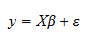## OLS estimator

The OLS estimatoris the solution to the minimization problem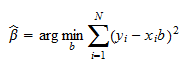Whenhas full rank, the solution is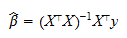## Variance of the OLS estimator

Under certain conditions, the covariance matrix of the OLS estimator is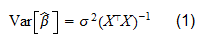whereis the variance offor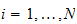.

In particular, this formula for the covariance matrix holds exactly in the normal linear regression model and asymptotically under the conditions stated in the lecture on the properties of the OLS estimator.

## Perfect multicollinearity

The most extreme case is that of perfect multicollinearity, in which at least one regressor can be expressed as a linear combination of other regressors. In this case, one of the columns ofcan be written as a linear combination of the other columns. As a consequence,is not full-rank and, by some elementary results on matrix products and ranks, the rank of the productis less than, so thatis not invertible. Thus, the formula for the OLS estimator cannot even be computed.

Roughly speaking, trying to invert a rank deficient matrix is like trying to compute the reciprocal of zero. In the case of perfect multicollinearity, we try to compute the variances in equation (1), and we incur into a division-by-zero problem: we divideby zero and, as a consequence, the variances of the regression coefficients (the diagonal elements of) go to infinity.

## Non-perfect multicollinearity

When one of the regressors is highly correlated with, but not equal to a linear combination of other regressors, then we say that the regression suffers from multicollinearity, although the multicollinearity is not perfect.

In such a case the design matrix is full-rank, but it is not very far from being rank-deficient.

Continuing with the division-by-zero analogy above, when multicollinearity is not perfect, we are dividingin equation (1) by a number that is very small, so that the variances of the regression coefficients are very large.

## Variance inflation factor

Ho do we measure the degree of multicollinearity? How do we make the definition of non-perfect multicollinearity above more precise?

The degree of multicollinearity is usually measured separately for each regressor(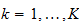), by comparing two quantities:

1. the variance that(the OLS estimate of the coefficient ofin the regression being estimated) would have ifwas uncorrelated with all the other regressors;

2. the actual variance of.

The ratio between these two quantities (actual/hypothetical variance) is called variance inflation factor (VIF). The VIF measures by how much the linear correlation of a given regressor with the other regressors increases the variance of its coefficient estimate with respect to the baseline case of no correlation.

It can be proved that the variance inflation factor for the coefficientis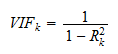whereis the R squared of a regression in whichis the dependent variable and() are the dependent variables.

If the regressor being examined is highly correlated with a linear combination of the other regressors, thenis close to 1 and the variance inflation factor is large. In the limit, whentends to 1, that is, in the case of perfect multicollinearity examined above,tends to infinity.

Although no rule of thumb is perfect (see O'Brien 2007), values of the VIF above 10 (i.e.,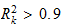) are often considered an indication that it might be worthwhile to adopt a remedy to reduce multicollinearity (see below).

## Numerical issues

There is an important and often overlooked issue about multicollinearity: whenis close to being singular, the result of calculating the inverse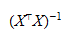on a computer can be severely biased by numerical error.

This is very important: even if we are comfortable with a high VIF and we are willing to tolerate the fact that the linear correlation among the regressors is inflating the variance of our OLS estimates, we should nonetheless check that multicollinearity is not a potential source of large numerical errors. In the next section we show how to do this check.

## Condition number

The condition number is the statistic most commonly used to check whether the inversion ofmay cause numerical problems.

Here we provide an intuitive introduction to the concept of condition number, but see Brandimarte (2007) for a formal but easy-to-understand introduction.

Consider the OLS estimateSince computers perform finite-precision arithmetic, they introduce round-off errors in the computation of products and additions such as those in the matrix product. In turn, these round-off errors are amplified (or dampened) when we multiplyby the inverse. By how much are the errors amplified? The condition number oftells us by how much they are amplified in the worst possible case. So, for example, if the condition number equals 100, then there are cases in which the round-off error in the computation ofis amplified 100-fold when multiplying it by.

While there are no precise rules, some authors (e.g., Greene 2000) suggest that multicollinearity is likely to cause numerical problems if the condition number ofis greater than 20.

Most statistical packages have built-in functions to compute the condition number of a matrix. For details on the computation of the condition number, see Brandimarte (2007).

## Remedies

What do we do to deal with multicollinearity?

### Remedies to perfect multicollinearity

In the case of perfect multicollinearity, at least one regressor is a linear combination of the other regressors. In other words, it is redundant and it does not add any information. Then the remedy is to simply drop it from the regression.

### Remedies to non-perfect multicollinearity

When multicollinearity is not perfect, the following remedies may be considered:

• increase the sample size; by doing so, the variance of the OLS estimates tends to decrease, even if it is inflated by high correlation among regressors; furthermore, a larger sample size decreases over-fitting and tends to reduce(see this lecture for a discussion of the impact of over-fitting on the R squared of a linear regression);

• drop one or more regressors that have a high VIF if they are not deemed to be essential;

• replace highly correlated regressors with a linear combination of them;

• use regularization methods such as ridge and lasso;

• use Bayesian regression; if multicollinearity prevents a regression coefficient from being estimated precisely, then the prior on that coefficient will help to reduce its posterior variance.

## References

Brandimarte, P. (2006) Numerical Methods in Finance and Economics: A MATLAB-Based Introduction, 2nd Edition, Wiley Interscience.

Greene, W.H. (2002) Econometric analysis, 5th edition. Prentice Hall.

O'Brien, R. (2007) A Caution Regarding Rules of Thumb for Variance Inflation Factors, Quality & Quantity, 41, 673-690.

The book

Most of the learning materials found on this website are now available in a traditional textbook format.

Glossary entries
Share